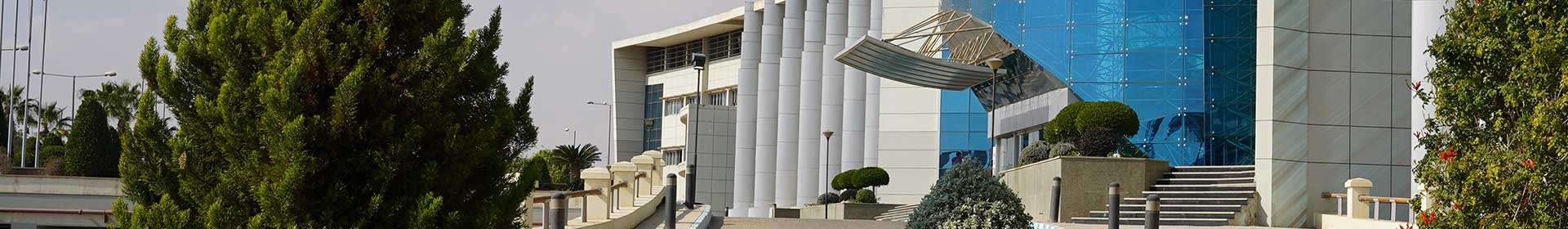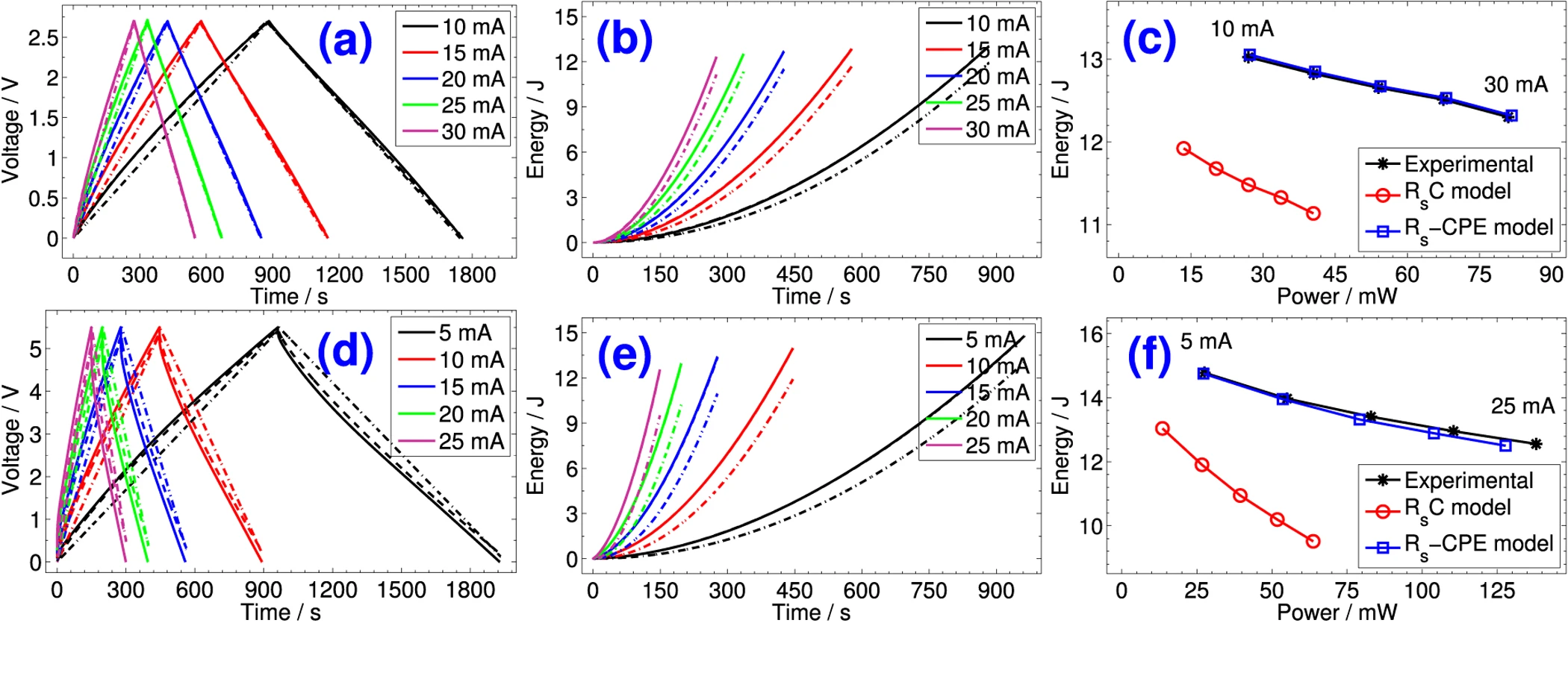Journal

# Reevaluation of Performance of Electric Double-layer Capacitors from Constant-current Charge/Discharge and Cyclic Voltammetry

By
Allagui A.
Freeborn T.J.
Elwakil A.S.
Maundy B.J.

The electric characteristics of electric-double layer capacitors (EDLCs) are determined by their capacitance which is usually measured in the time domain from constant-current charging/discharging and cyclic voltammetry tests, and from the frequency domain using nonlinear least-squares fitting of spectral impedance. The time-voltage and current-voltage profiles from the first two techniques are commonly treated by assuming ideal S s C behavior in spite of the nonlinear response of the device, which in turn provides inaccurate values for its characteristic metrics. In this paper we revisit the calculation of capacitance, power and energy of EDLCs from the time domain constant-current step response and linear voltage waveform, under the assumption that the device behaves as an equivalent fractional-order circuit consisting of a resistance R s in series with a constant phase element (CPE(Q, α), with Q being a pseudocapacitance and α a dispersion coefficient). In particular, we show with the derived (R s, Q, α)-based expressions, that the corresponding nonlinear effects in voltage-time and current-voltage can be encompassed through nonlinear terms function of the coefficient α, which is not possible with the classical R s C model. We validate our formulae with the experimental measurements of different EDLCs. © 2016 The Author(s).